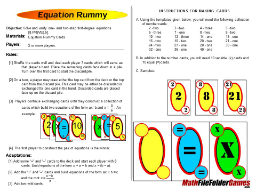# Equation Rummy: Solving Equations Game

Download this Game FREE and File it Away for Later! (NO EMAIL Opt-in Required)

Students will need to think carefully about the cards they decide to keep, since they are not only selecting the numbers which will work to yield a positive answer for their result, they will also be selecting the appropriate symbol cards to give their equation meaning.

This game provides an excellent way to model simple equations. It’s perfect as an introduction to equation solving for a 6th or 7th grade class.

Common Core Mathematical Standard

6.EE Reason about and solve one-variable equations and inequalities.
7. Solve real-world and mathematical problems by writing and solving equations of the form x + p = q and px = q for cases in which p, q and x are all nonnegative rational numbers.A full color version (green) and a less color more white space version of this game is included in this free download!Get the above math game PLUS 41 more fun middle school (& upper elementary) math games to use with your students.